Hypothesis

A hypothesis is a tentative relationship between two or more variables. These variables are related to various aspects of the research inquiry.

A hypothesis is a testable prediction. It can be a false or a true statement that is tested in the research to check its authenticity.

A researcher has to explore various aspects of the research topic. Thus, a researcher assumes some possible relationship between variables related to each aspect of the research topic. Testing these relationships helps the researcher to explore different aspects of the research. Such a possible relationship between variables is called a hypothesis.

Hypotheses are purposefully formulated by a researcher because it is difficult to start research without a valid foundation. Hence, the researcher builds logical relationships between or among the variables of the research. As these variables are related to the theme of the research, the relationships among them serve as a foundation for the research. These logical relationships or testable assumptions give a direction to the researcher to start working on the research.

For instance, a researcher working on the topic ‘Discrimination against Women in a Rural Society’ will construct the following hypotheses:

• Higher the illiteracy in society, higher will be the discrimination against women.
• Higher the patriarchy in society, higher will be the discrimination against women.
• Higher the traditional practices in a society, higher will be discrimination against women.

Similarly, a researcher working on the topic ‘Extent of Use of Family-Planning Practice in an Area’ may devise the following hypotheses:

• Higher the standard of education, higher will be the use of the family-planning practice.
• Higher the availability of family-planning services, higher will be the use of family planning practice.
• Higher the standards of living, higher will be the use of the family-planning practice.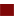Characteristics of Hypothesis

1. Empirically Testable
2. Simple and Clear
3. Specific and relevant to theme of research
4. Predictable
5. ManageableImportance of Hypothesis

1. It gives a direction to the research.
2. It makes it easier to explore various aspects of the research.
3. It specifies the focus of the researcher, because, without hypotheses, the research may also focus on the unnecessary and unintended aspects of the research.
4. It helps in devising research techniques.
5. It prevents researcher from blind research.
6. It ensures the accuracy and precision of the findings of the research.
7. It saves resources – time, money and energy – because, without hypotheses, the researcher may utilize these resources on the unnecessary dimension of the study.TYPES OF HYPOTHESIS

The types of hypotheses are as follows:

1. Simple Hypothesis
2. Complex Hypothesis
3. Working or Research Hypothesis
4. Null Hypothesis
5. Alternative Hypothesis
6. Logical Hypothesis
7. Statistical Hypothesis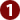Simple Hypothesis

A simple hypothesis is a hypothesis that reflects a relationship between two variables – independent and dependent variable.

Examples:

• Higher the unemployment, higher would be the rate of crime in society.
• Lower the use of fertilizers, lower would be agricultural productivity.
• Higher the poverty in a society, higher would be the rate of crime.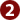Complex Hypothesis

A complex hypothesis is a hypothesis that reflects a relationship among more than two variables.

Examples:

1. Higher the poverty, higher the illiteracy in society, higher will be the rate of crime (three variables – two independent variables and one dependent variable).
2. Higher the use of fertilizers, improved seeds, and modern equipments, higher would be the agricultural productivity (Four variable – three independent variables and one dependent variable).
3. Higher the illiteracy in a society higher will be poverty and crime rate. (Three variables – one independent variable and two dependent variables)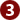Working Hypothesis

A hypothesis, that is accepted to put to test and work on in the research, is called a working hypothesis. It is a hypothesis that is assumed to be suitable to explain certain facts and relationship of phenomena. It is expected that this hypothesis would generate a productive theory and is accepted to put to test for investigation.

It can simply be any hypothesis that is initially accepted for working on in the research.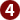Alternative Hypothesis

If the working hypothesis is proved wrong or rejected, another hypothesis (to replace the working hypothesis) is formulated to be tested to explore the desired aspect of research – this is known as an alternate hypothesis.

As the name mentions, it is an alternate assumption (or relationship) which is adopted after the working hypothesis fails to generate the required theory. Alternative Hypothesis is denoted by H1.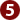Null Hypothesis

A null hypothesis is a hypothesis that expresses no relationship between variables. It negates the association between variables.

Examples:

• Poverty has nothing to do with the rate of crime in a society.
• Illiteracy has nothing to do with the rate of unemployment in society.

A null hypothesis has its purpose. A null hypothesis is made with an intention where the researcher intentionally wants to disapprove, reject or nullify the null hypothesis, and thus, to confirm a relationship between the variables. A null hypothesis is usually made for as a reverse strategy – to prove it wrong in order to confirm that there does exist a relationship between the variables. A null hypothesis is denoted by HO.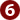Statistical Hypothesis

A hypothesis, that can be verified statistically, is known as a statistical hypothesis.

It can be any hypothesis that has the quality of being verified statistically. It means that it can be tested with the help of quantitative techniques. It can also be said that the variables in a statistical hypothesis are quantifiable or can be transformed into its quantifiable indicators to test it statistically.Logical Hypothesis

A hypothesis, that can be verified logically, is known as a logical hypothesis.

It is a hypothesis expressing a relationship whose inter-links can be connected on the basis of logical explanations in order to verify it. It can be verified with the help of logical evidence. It does not necessarily mean that a logical hypothesis cannot be verified with the help of statistical techniques. It may or may not be statistically verifiable, but it seems so plausible in the light of the logical explanations that these logical considerations suffice to verify it.